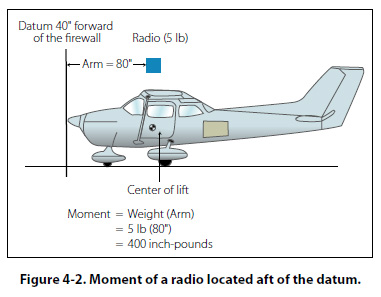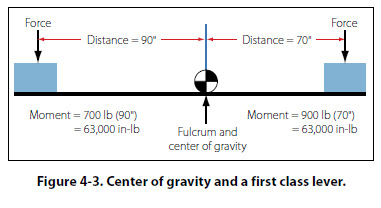Moment A moment is the product of a weight multiplied by its arm. The moment for a piece of equipment is in fact a torque value, measured in units of inch-pounds (in-lb). To obtain the moment of an item with respect to the datum, multiply the weight of the item by its horizontal distance from the datum. Likewise, the moment of an item with respect to the center of gravity (CG) of an aircraft can be computed by multiplying its weight by the horizontal distance from the CG. A 5 lb radio located 80" from the datum would have a moment of 400 inch-pounds (in-lb) (5 lb × 8"). Whether the value of 400 in-lb is preceded by a positive (+) or negative (-) sign depends on whether the moment is the result of a weight being removed or added and its location in relation to the datum. This situation is shown in Figure 4-2, where the moment ends up being a positive number because the weight and arm are both positive.The algebraic sign of the moment, based on the datum location and whether weight is being installed or removed, would be as follows: Weight being added aft of the datum produces a positive moment (+ weight, + arm). Weight being added forward of the datum produces a negative moment (+ weight, - arm). Weight being removed aft of the datum produces a negative moment (- weight, + arm). Weight being removed forward of the datum produces a positive moment (- weight, - arm) When dealing with positive and negative numbers, remember that the product of like signs produces a positive answer and the product of unlike signs produces a negative answer. Center of Gravity The center of gravity (CG) of an aircraft is a point about which the nose heavy and tail heavy moments are exactly equal in magnitude. It is the balance point for the aircraft. An aircraft suspended from this point would have no tendency to rotate in either a nose-up or nose-down attitude. It is the point about which the weight of an airplane or any object is concentrated.Figure 4-3 shows a first class lever with the pivot point (fulcrum) located at the center of gravity for the lever. Even though the weights on either side of the fulcrum are not equal, and the distances from each weight to the fulcrum are not equal, the product of the weights and arms (moments) are equal, and that is what produces a balanced condition. Maximum Weight The maximum weight is the maximum authorized weight of the aircraft and its contents, and is indicated in the Aircraft Specifications or Type Certificate Data Sheet. For many aircraft, there are variations to the maximum allowable weight, depending on the purpose and conditions under which the aircraft is to be flown. For example, a certain aircraft may be allowed a maximum gross weight of 2,750 lb when flown in the normal category, but when flown in the utility category, which allows for limited aerobatics, the same aircraft’s maximum allowable gross weight might only be 2,175 lb. There are other variations when dealing with the concept of maximum weight, as follows: Maximum Ramp Weight—the heaviest weight to which an aircraft can be loaded while it is sitting on the ground. This is sometimes referred to as the maximum taxi weight. Maximum Takeoff Weight—the heaviest weight an aircraft can have when it starts the takeoff roll. The difference between this weight and the maximum ramp weight would equal the weight of the fuel that would be consumed prior to takeoff. Maximum Landing Weight—the heaviest weight an aircraft can have when it lands. For large wide body commercial airplanes, it can be 100,000 lb less than maximum takeoff weight, or even more. Maximum Zero Fuel Weight—the heaviest weight an aircraft can be loaded to without having any usable fuel in the fuel tanks. Any weight loaded above this value must be in the form of fuel.
 ©AvStop Online Magazine                                                                                                                                                      Contact Us              Return To Books